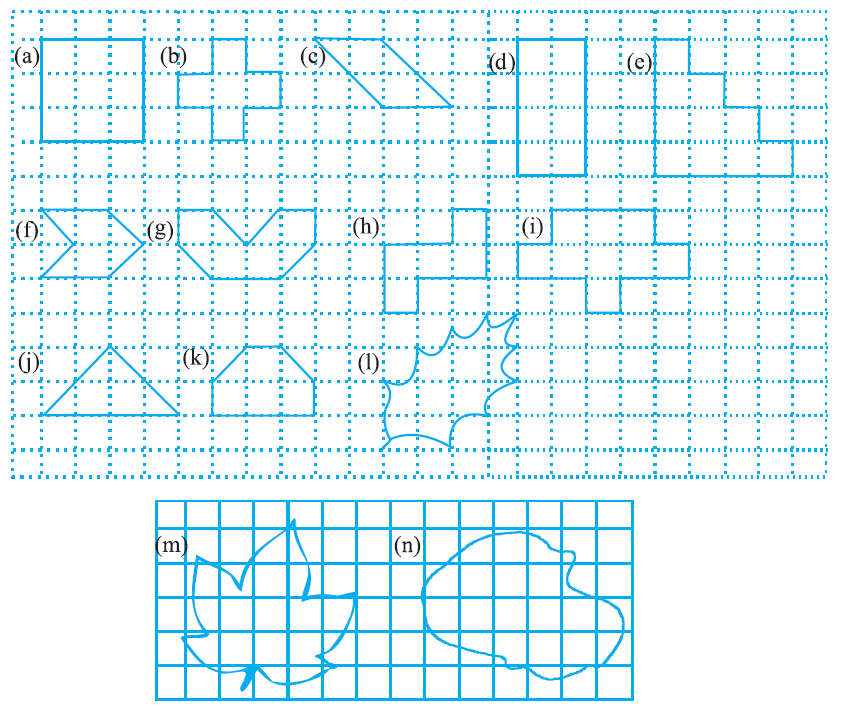# vs.eyeandcontacts.com

## Chapter 10 Mensuration Exercise 10.2

Question 1: Find the areas of the following figures by counting square:a) The figure contains only 9 fully filled squares. Therefore, the area of this figure will be 9 square units.

b) The figure contains only 5 fully filled squares. Therefore, the area of this figure will be 5 square units.

c) The figure contains 2 fully filled squares and 4 half-filled squares. Therefore, the area of this figure will be 4 square units.

d) The figure contains only 8 fully filled squares. Therefore, the area of this figure will be 8 square units.

e) The figure contains only 10 fully filled squares. Therefore, the area of this figure will be 10 square units.

f) The figure contains only 2 fully filled squares and 4 half-filled squares. Therefore, the area of this figure will be 4 square units.

g) The figure contains 4 fully filled squares and 4 half-filled squares. Therefore, the area of this figure will be 6 square units.

h) The figure contains 5 fully filled squares. Therefore, the area of this figure will be 5 square units.

i) The figure contains 9 fully filled squares. Therefore, the area of this figure will be 9 square units.

j) The figure contains 2 fully filled squares and 4 half-filled squares. Therefore, the area of this figure will be 4 square units.

k) The figure contains 4 fully filled squares and 2 half-filled squares. Therefore, the area of this figure will be 5 square units.

l)

 Covered Area Number Area estimate (square units) Fully filled squares 2 2 Half-filled squares – – More than half filled squares 6 6 Less than half filled squares 6 0

m)
 Covered Area Number Area estimate (square units) Fully filled squares 5 5 Half-filled squares – – More than half filled squares 9 9 Less than half filled squares 12 0

n)
 Covered Area Number Area estimate (square units) Fully filled squares 8 8 Half-filled squares – – More than half filled squares 10 10 Less than half filled squares 9 0